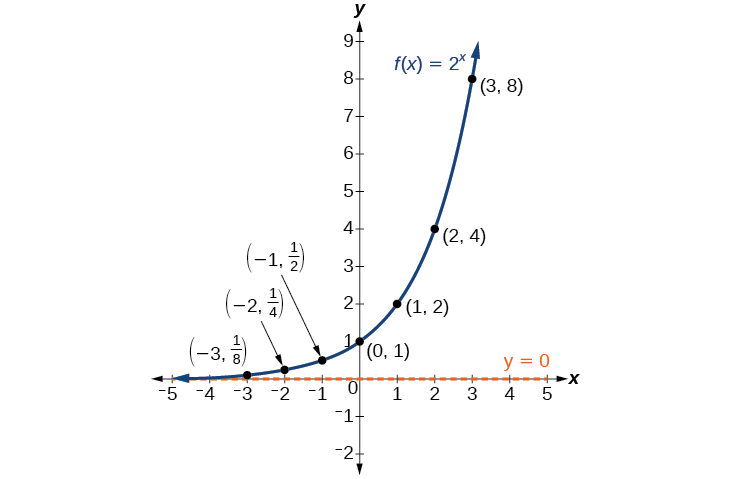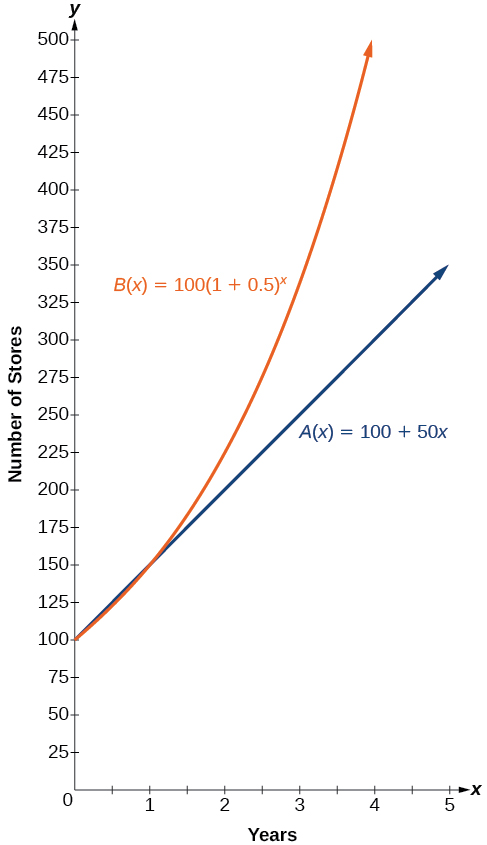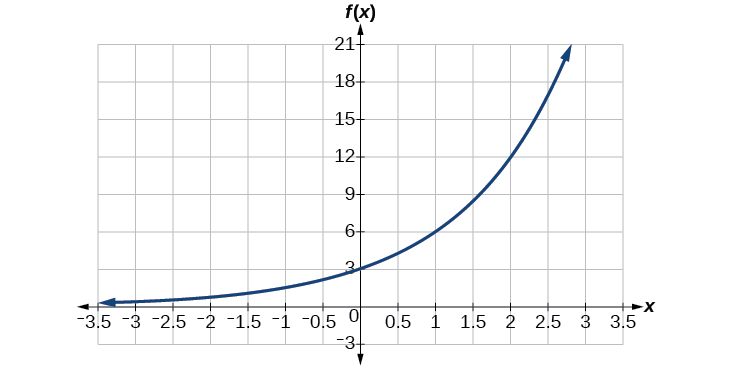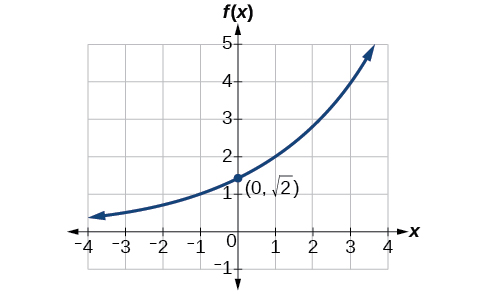## 2.2 Exponential Functions

### Learning Objectives

In this section, you will:

• Compare exponential functions to linear functions.
• Evaluate exponential functions.
• Determine the characteristics of exponential functions.
• Find the equation of an exponential function.
• Find equations that use continuous growth and decay rates.

India is the second most populous country in the world with a population of about 1.37 billion people in 2019. The population is growing at a rate of about 1.08% each year. If this rate continues, the population of India will exceed China’s population by the year 2031.  When populations grow rapidly, we often say that the growth is “exponential,” meaning that something is growing very rapidly. To a mathematician, however, the term exponential growth has a very specific meaning. In this section, we will take a look at exponential functions, which model this kind of rapid growth.

### Identifying Exponential Functions

When exploring linear growth, we observed a constant rate of change—a constant number by which the output increased for each unit increase in the input. For example, in the equation $f\left(x\right)=3x+4,$ the slope tells us the output increases by 3 each time the input increases by 1. The scenario in the India population example is different because we have a percent change per unit time (rather than a constant change) in the number of people.

#### Defining an Exponential Function

A study found that the percent of the population who are vegans in the United States doubled from 2009 to 2011. In 2011, 2.5% of the population was vegan, adhering to a diet that does not include any animal products—no meat, poultry, fish, dairy, or eggs. If this rate continues, vegans will make up 10% of the U.S. population in 2015, 40% in 2019, and 80% in 2021.

What exactly does it mean to grow exponentially? What does the word double have in common with percent increase? People toss these words around errantly. Are these words used correctly? The words certainly appear frequently in the media.

• Percent change refers to a change based on a percent of the original amount.
• Exponential growth refers to an increase based on a constant multiplicative rate of change over equal increments of time, that is, a percent increase of the original amount over time.
• Exponential decay refers to a decrease based on a constant multiplicative rate of change over equal increments of time, that is, a percent decrease of the original amount over time.

For us to gain a clear understanding of exponential growth, let us contrast exponential growth with linear growth. We will construct two functions. The first function is exponential. We will start with an input of 0, and increase each input by 1. We will double the corresponding consecutive outputs. The second function is linear. We will start with an input of 0, and increase each input by 1. We will add 2 to the corresponding consecutive outputs. See Table 1.

Table 1
$x$ $f\left(x\right)={2}^{x}$ $g\left(x\right)=2x$
0 1 0
1 2 2
2 4 4
3 8 6
4 16 8
5 32 10
6 64 12

From Table 1 we can infer that for these two functions, exponential growth dominates linear growth.

• Exponential growth refers to the original value from the range increases by the same percentage over equal increments found in the domain. Another way to say this that there is a constant multiplier over equal increments in the domain.
• Linear growth refers to the original value from the range increases by the same amount over equal increments found in the domain.

Apparently, the difference between “the same percentage” and “the same amount” is quite significant. For exponential growth, over equal increments, the constant multiplicative rate of change resulted in doubling the output whenever the input increased by one. For linear growth, the constant additive rate of change over equal increments resulted in adding 2 to the output whenever the input was increased by one.

The general form of the exponential function is $f\left(x\right)=a{b}^{x},$ where $a$ is any nonzero number, and $b$ is a positive real number not equal to 1.

• If $b>1,$ the function grows at a rate proportional to its size.
• If $0<b<1,$ the function decays at a rate proportional to its size.

Let’s look at the function $f\left(x\right)={2}^{x}$ from our example. We will create a table to determine the corresponding outputs over an interval in the domain from $-3$ to $3.$ See Table 2.

 $x$ $-3$ $-2$ $-1$ $0$ $1$ $2$ $3$ $f\left(x\right)={2}^{x}$ ${2}^{-3}=\frac{1}{8}$ ${2}^{-2}=\frac{1}{4}$ ${2}^{-1}=\frac{1}{2}$ ${2}^{0}=1$ ${2}^{1}=2$ ${2}^{2}=4$ ${2}^{3}=8$

Let us examine the graph of $f$ by plotting the ordered pairs we observe on the table, and then make a few observations. See Figure 1.Figure 1.

Let’s define the behavior of the graph of the exponential function $f\left(x\right)={2}^{x}$ and highlight some its key characteristics.

• The domain is $\left(-\infty ,\infty \right).$
• The range is $\left(0,\infty \right).$
• As x gets larger and larger so does $f\left(x\right).$
• The graph of $f\left(x\right)$ will never touch the x-axis because base two raised to any exponent never has the result of zero.
• $f\left(x\right)$ is always increasing.
• The vertical intercept is $\left(0,1\right)$.

### Exponential Function

For any real number $x,$ an exponential function is a function with the form

$f\left(x\right)=a{b}^{x}$

where

• $a$ is a non-zero real number called the initial value or initial condition and
• $b$ is any positive real number such that $b\ne 1.$
• The domain of $f$ is all real numbers.
• The range of $f$ is all positive real numbers if $a>0.$
• The range of $f$ is all negative real numbers if $a<0.$
• The vertical intercept is $\left(0,a\right).$

### Example 1:  Identifying Exponential Functions

Which of the following equations are not exponential functions?

• $f\left(x\right)={4}^{3\left(x-2\right)}$
• $g\left(x\right)={x}^{3}$
• $h\left(x\right)={\left(\frac{1}{3}\right)}^{x}$
• $j\left(x\right)={\left(-2\right)}^{x}$

### Try it #1

Which of the following equations represent exponential functions?

• $f\left(x\right)=2{x}^{2}-3x+1$
• $g\left(x\right)={0.875}^{x}$
• $h\left(x\right)=1.75x+2$
• $j\left(x\right)={1095.6}^{-2x}$

### Writing Formulas for Exponential Functions and Evaluating Them

Recall that the base of an exponential function must be a positive real number other than $1.$  Why do we limit the base $b$ to positive values? To ensure that the outputs will be real numbers. Observe what happens if the base is not positive:

• Let $b=-9$ and $x=\frac{1}{2}.$ Then $f\left(\frac{1}{2}\right)={\left(-9\right)}^{\frac{1}{2}}=\sqrt[\leftroot{1}\uproot{2} ]{-9},$ which is not a real number.

Why do we limit the base to positive values other than $1?$  Because base $1$ results in the constant function. Observe what happens if the base is $1:$

• Let $b=1.$ Then $f\left(x\right)={1}^{x}=1$ for any value of $x.$

To evaluate an exponential function with the form $f\left(x\right)={b}^{x},$ we simply substitute $x$ with the given value, and calculate the resulting power. For example:

Let $f\left(x\right)={2}^{x}.$ What is $f\left(3\right)?$

\begin{align*}f\left(x\right)&={2}^{x}&& \hfill \\ f\left(3\right)&={2}^{3}&& \text{Substitute }x=3.\\ \hfill &=8&& \text{Evaluate the power}\text{.}\end{align*}

To evaluate an exponential function with a form other than the basic form, it is important to follow the order of operations. For example:

Let $f\left(x\right)=30{\left(2\right)}^{x}.$ What is $f\left(3\right)?$

\begin{align*}f\left(x\right)&=30{\left(2\right)}^{x}&& \hfill \\ f\left(3\right)&=30{\left(2\right)}^{3}&& \textrm{Substitute }x=3.\hfill \\ \hfill &=30\left(8\right)\text{ }&& \textrm{Simplify the power first}\text{.}\hfill \\ \hfill &=240&& \textrm{Multiply}\text{.}\hfill \end{align*}

Note that if the order of operations were not followed, the result would be incorrect:

$f\left(3\right)=30{\left(2\right)}^{3}\ne {60}^{3}=216,000$

### Example 2:  Evaluating Exponential Functions

Let $f\left(x\right)=5{\left(3\right)}^{x+1}.$ Evaluate $f\left(2\right)$ without using a calculator.

### Try it #2

Let $f\left(x\right)=8{\left(1.2\right)}^{x-5}.$ Evaluate $f\left(3\right)$ using a calculator. Round to four decimal places.

#### Defining Exponential Growth and Decay

Because the output of exponential functions increases very rapidly, the term “exponential growth” is often used in everyday language to describe anything that grows or increases rapidly. However, exponential growth can be defined more precisely in a mathematical sense. If the growth rate is proportional to the amount present, the function models exponential growth or decay.

### Exponential Growth or Decay

A function that models exponential growth or decay grows by a rate proportional to the amount present. For any real number $x$ and any positive real numbers $a$ and $b$ such that $b\ne 1,$ an exponential growth function has the form

$f\left(x\right)=a{b}^{x}=a\left(1+r\right)^x$

where

• $a$ is the initial or starting value of the function.
• $b=1+r$ is the growth factor or growth multiplier per unit $x$.
• $r = b - 1$ is the percent increase or decrease expressed as a decimal.

In more general terms, we have an exponential function, in which a constant base is raised to a variable exponent. To differentiate between linear and exponential functions, let’s consider two companies, A and B. Company A has 100 stores and expands by opening 50 new stores a year, so its growth can be represented by the function $A\left(x\right)=100+50x.$ Company B has 100 stores and expands by increasing the number of stores by 50% each year, so its growth can be represented by the function $B\left(x\right)=100{\left(1+0.5\right)}^{x}.$

A few years of growth for these companies are illustrated in Table 3.

Table 3
Year, $x$ Stores, Company A Stores, Company B
$0$ $100+50\left(0\right)=100$ Starting with 100 each $100{\left(1+0.5\right)}^{0}=100$
$1$ $100+50\left(1\right)=150$ Both grow by 50 stores in the first year. $100{\left(1+0.5\right)}^{1}=150$
$2$ $100+50\left(2\right)=200$ Company A grows by 50 stores and company B by 75 stores. $100{\left(1+0.5\right)}^{2}=225$
$3$ $100+50\left(3\right)=250$ Company A grows by 50 stores and company B by 112.5 stores. $100{\left(1+0.5\right)}^{3}=337.5$
$x$ $A\left(x\right)=100+50x$ $B\left(x\right)=100{\left(1+0.5\right)}^{x}$

The graphs comparing the number of stores for each company over a five-year period are shown in Figure 2. We can see that, with exponential growth, the number of stores increases much more rapidly than with linear growth.Figure 2.

Notice that the contextual domain for both functions is $\left[0,\infty \right),$ and the range for both functions is $\left[100,\infty \right).$ After year 1, Company B always has more stores than Company A.

Now we will turn our attention to the function representing the number of stores for Company B,

$B\left(x\right)=100{\left(1+0.5\right)}^{x}.$

In this exponential function, 100 represents the initial number of stores, 0.50 represents the growth rate, $r,$ and $b=1+0.5=1.5$ represents the growth factor. Generalizing further, we can write this function as

$B\left(x\right)=100{\left(1.5\right)}^{x}.$

### Example 3:  Evaluating a Real-World Exponential Model

At the beginning of this section, we learned that the population of India was about 1.37 billion in the year 2019, with an annual growth rate of about 1.08%.  Let $t$ be the number of years since 2019. Find an exponential function to model this growth.  Then, to the nearest thousandth, determine what will the population of India be in 2031.

### Example 4:  Real World Exponential Models

Bismuth-210 is an isotope that radioactively decays by about 13% each day, meaning 13% of the remaining Bismuth-210 transforms into another atom (polonium-210 in this case) each day. If you begin with 100 mg of Bismuth-210, how much remains after one week?

### Try it #3

The population of China was about 1.39 billion in the year 2013, with an annual growth rate of about 0.6%. Find an exponential function that models this situation where $t$ represents the number of years since 2013.  To the nearest thousandth, what will the population of China be for the year 2031?

### Try It #4

A population of 1000 animals is decreasing 3% each year. Find the population in 30 years.

### Finding Equations of Exponential Functions From Data

In the previous examples, we were given an exponential growth or decay rate, which we used to find a formula to model the situation and then evaluated for a given input. Sometimes we are given data points for an exponential function and we must use them to first find the growth factor and then the formula for the function before we can answer questions about the situation.

### How To

Given two data points with the initial value known, write an exponential model.

1. Identify the initial value from the data point of the form $\left(0,a\right).$ Then $a$ is the initial value.
2. Using $a,$ substitute the second point into the equation $f\left(x\right)=a{\left(b\right)}^{x},$ and solve for $b.$  To do this, divide both sides by $a$ and then raise both sides to the appropriate power to solve the equation.
3. Write the equation using the values you found for $a$ and $b$ in the form $f\left(x\right)=a{\left(b\right)}^{x}.$

### Example 5:  Writing an Exponential Model When the Initial Value Is Known

In 2006, 80 deer were introduced into a wildlife refuge. By 2012, the population had grown to 180 deer. The population was growing exponentially. Write an algebraic function $N\left(t\right)$ representing the population $\left(N\right)$ of deer over time $t.$

### Try it #5

A wolf population is growing exponentially. In 2011, 129 wolves were counted. By 2013, the population had reached 236 wolves. Let $t=0$ represent the year 2011.   What two points can be used to derive an exponential equation modeling this situation? Write the equation representing the population, $N\text{, }$of wolves over time $t.$

### How To

Given two data points, write an exponential model with the initial value unknown.

Method 1: Substitution

1. Substitute both points into two equations with the form $f\left(x\right)=a{\left(b\right)}^{x}.$
2. Solve the first equation for $a$ in terms of $b.$
3. Substitute this expression for $a$ into the second equation and then solve for $b.$
4. Once you have found $b,$ substitute a data point and $b$ into the general equation and solve for $a$.
5. Using the $a$ and $b$ found in the steps above, write the exponential function in the form $f\left(x\right)=a{\left(b\right)}^{x}.$

Method 2: Ratios

1. Choose two points to work with:  $\left(c, f\left(c\right)\right)$ and $\left(d, f\left(d\right)\right).$ Order your points so $c < d.$
2. Set up your ratio: $\frac{f\left(d\right)}{f\left(c\right)}=\frac{ab^d}{ab^c}.$ Note that the values on the left are gotten from your output values of the data points and the values on the right come from the general equation with $c$ and $d$ filled in.
3. Simplify. Note that $\frac{a}{a}=1$ so essentially they always cancel and you will always find $b$ first.  Remember that $\frac{b^d}{b^c}=b^{d-c}.$ You will then need to find the (d-c)th root of both sides.
4. Once you have found $b,$ substitute a data point and $b$ into the general equation and solve for $a$.
5. Write your equation using the values of $a$ and $b$ in the form $f\left(x\right)=a{\left(b\right)}^{x}.$

### Example 6:  Writing an Exponential Model When the Initial Value is Not Known

Find an exponential function that passes through the points $\left(-2,6\right)$ and $\left(2,1\right).$

### Try it #6

Given the two points $\left(1,3\right)$ and $\left(2,4.5\right),$ find the equation of the exponential function that passes through these two points.

### Q&A

Do two points always determine a unique exponential function?

Yes, provided the two points are either both above the x-axis or both below the x-axis and have different x-coordinates. But keep in mind that we also need to know that the graph is, in fact, an exponential function. Not every graph that looks exponential really is exponential. We need to know the graph is based on a model that shows the same percent growth with each unit increase in $x,$ which in many real world cases involves time.

### How To

Given a graph or a table of an exponential function, write its equation.

1. First, identify two points on the graph or table. Choose the vertical intercept as one of the two points whenever possible. Try to choose points that are as far apart as possible to reduce round-off error.
2. Use one of the methods for finding an equation given two points above.

### Example 7:  Writing an Exponential Function Given Its Graph

Find an equation for the exponential function graphed in Figure 5.Figure 5.

### Try it #7

Find an equation for the exponential function graphed in Figure 6.Figure 6.

### Example 8: Writing an Exponential Function Given a Table of Values

Find an equation for the exponential function given by Table 4.

 $x$ 1 3 6 8 12 $f\left(x\right)$ 4.8 6.912 11.9439 17.1993 35.6644

### Applying the Compound-Interest Formula

Savings instruments in which earnings are continually reinvested, such as mutual funds and retirement accounts, use compound interest. The term compounding refers to interest earned not only on the original value, but on the accumulated value of the account.

The annual percentage rate (APR) of an account, also called the nominal rate, is the yearly interest rate earned by an investment account. The term nominal is used when the compounding occurs a number of times other than once per year. In fact, when interest is compounded more than once a year, the effective interest rate ends up being greater than the nominal rate! This is a powerful tool for investing.

We can calculate the compound interest using the compound interest formula, which is an exponential function of the variables time $t,$ principal $P,$ APR $r,$ and number of compounding periods in a year $n:$

$A\left(t\right)=P{\left(1+\frac{r}{n}\right)}^{nt}.$

For example, observe Table 5, which shows the result of investing $1,000 at 10% for one year. Notice how the value of the account increases as the compounding frequency increases. Table 5 Frequency Value after 1 year Annually$1100
Semiannually $1102.50 Quarterly$1103.81
Monthly $1104.71 Daily$1105.16

### The Compound Interest Formula

Compound interest can be calculated using the formula

$A\left(t\right)=P{\left(1+\frac{r}{n}\right)}^{nt}$

where

• $A\left(t\right)$ is the account value,
• $t$ is measured in years,
• $P$ is the starting amount of the account, often called the principal, or more generally present value,
• $r$ is the annual percentage rate (APR) expressed as a decimal, and
• $n$ is the number of compounding periods in one year.

A 529 Plan is a college-savings plan that allows relatives to invest money to pay for a child’s future college tuition; the account grows tax-free. Lily wants to set up a 529 account for her new granddaughter and wants the account to grow to $40,000 over 18 years. She believes the account will earn 6% compounded semi-annually (twice a year). To the nearest dollar, how much will Lily need to invest in the account now? ### Try it #9 Refer to Example 10. To the nearest dollar, how much would Lily need to invest if the account is compounded quarterly? ### Evaluating Functions with Base e As we saw earlier, the amount earned on an account increases as the compounding frequency increases. Table 6 shows that the increase from annual to semi-annual compounding is larger than the increase from monthly to daily compounding. This might lead us to ask whether this pattern will continue. Examine the value of$1 invested at 100% interest for 1 year, compounded at various frequencies, listed in Table 6.

Table 6
Frequency $A\left(n\right)={\left(1+\frac{1}{n}\right)}^{n}$ Value
Annually ${\left(1+\frac{1}{1}\right)}^{1}$ $2 Semiannually ${\left(1+\frac{1}{2}\right)}^{2}$$2.25
Quarterly ${\left(1+\frac{1}{4}\right)}^{4}$ $2.441406 Monthly ${\left(1+\frac{1}{12}\right)}^{12}$$2.613035
Daily ${\left(1+\frac{1}{365}\right)}^{365}$ $2.714567 Hourly ${\left(1+\frac{1}{\text{8760}}\right)}^{\text{8760}}$$2.718127
Once per minute ${\left(1+\frac{1}{\text{525600}}\right)}^{\text{525600}}$ $2.718279 Once per second ${\left(1+\frac{1}{31536000}\right)}^{31536000}$$2.718282

These values appear to be approaching a limit as $n$ increases without bound. In fact, as $n$ gets larger and larger, the expression ${\left(1+\frac{1}{n}\right)}^{n}$ approaches a number used so frequently in mathematics that it has its own name: the letter $e.$ This value is an irrational number, which means that its decimal expansion goes on forever without repeating. Its approximation to six decimal places is shown below.

### Euler’s Number

The letter e represents the irrational number ${\left(1+\frac{1}{n}\right)}^{n},$ as $n$ increases without bound.

The letter e is used as a base for many real-world exponential models. To work with base e, we use the approximation, $e\approx 2.718282.$ The constant was named by the Swiss mathematician Leonhard Euler (1707–1783) who first investigated and discovered many of its properties.

### Example 11:  Using a Calculator to Find Powers of e

Calculate ${e}^{3.14}.$ Round to five decimal places.

### Try it #10

Use a calculator to find ${e}^{-0.5}.$ Round to five decimal places.

### Investigating Continuous Growth

So far we have worked with rational bases for exponential functions. For many real-world phenomena, however, e is used as the base for exponential functions. Exponential models that use $e$ as the base are called continuous growth or decay models. We see these models in finance, computer science, and most of the sciences, such as physics, toxicology, and fluid dynamics.

### The Continuous Growth/Decay Formula

For all real numbers $t,$ and all positive numbers $a$ and $k,$ continuous growth or decay is represented by the formula

$A\left(t\right)=a{e}^{kt}$

where

• $a$ is the initial value,
• $k$ is the continuous rate per unit time, and
• $t$ is the elapsed time.

If $k>0$, then the formula represents continuous growth. If $k<0$, then the formula represents continuous decay.

### How To

Given the initial value, continuous rate of growth or decay, and time, solve a continuous growth or decay function.

1. Use the information in the problem to determine $a$, the initial value of the function.
2. Use the information in the problem to determine the growth rate $k.$
• If the problem refers to continuous growth, then $k>0.$
• If the problem refers to continuous decay, then $k<0.$
3. Use the information in the problem to determine the time $t.$
4. Substitute the given information into the continuous growth formula and simplify.

### Example 13: Calculating Continuous Decay

Radon-222 decays at a continuous rate of 17.3% per day. How much will 100 mg of Radon-222 decay to in 3 days?

### Try it #12

Using the data in Example 13, how much radon-222 will remain after one year?

### Media

Access these online resources for additional instruction and practice with exponential functions.

Key Equations

 definition of exponential growth or decay $f\left(x\right)=a{b}^{x},\text{ where }a>0,b>0,b\ne 1$ compound interest formula $\begin{array}{l}A\left(t\right)=P{\left(1+\frac{r}{n}\right)}^{nt} ,\text{ where}\hfill \\ A\left(t\right)\text{ is the account value at time }t\hfill \\ t\text{ is the number of years}\hfill \\ P\text{ is the initial investment, often called the principal}\hfill \\ r\text{ is the annual percentage rate (APR), or nominal rate}\hfill \\ n\text{ is the number of compounding periods in one year}\hfill \end{array}$ continuous growth formula $A\left(t\right)=a{e}^{kt},\text{ where}$ $t$ is the number of unit time periods of growth $a$ is the starting amount (in the continuous compounding formula $a$ may be replaced with P, the principal) $e$ is the mathematical constant,$\text{ }e\approx 2.718282$

### Key Concepts

• An exponential function is defined as a function with a positive constant other than $1$ raised to a variable exponent.
• A function is evaluated by solving at a specific value.
• An exponential model can be found when the growth rate and initial value are known.
• An exponential model can be found when the two data points from the model are known.
• An exponential model can be found using two data points from a graph or a table of the model.
• The value of an account at any time $t$ can be calculated using the compound interest formula when the principal, annual interest rate, and compounding periods are known.
• The initial investment of an account can be found using the compound interest formula when the value of the account, annual interest rate, compounding periods, and life span of the account are known.
• The number $e$ is a mathematical constant often used as the base of real world exponential growth and decay models. Its decimal approximation is $e\approx 2.718282.$
• Continuous growth or decay models are exponential models that use $e$ as the base. Continuous growth and decay models can be found when the initial value and continuous growth or decay rate are known.

### Glossary

annual percentage rate (APR)
the yearly interest rate earned by an investment account, also called nominal rate
compound interest
interest earned on the total balance, not just the principal
exponential growth
a model that grows by a rate proportional to the amount present
nominal rate
the yearly interest rate earned by an investment account, also called annual percentage rate